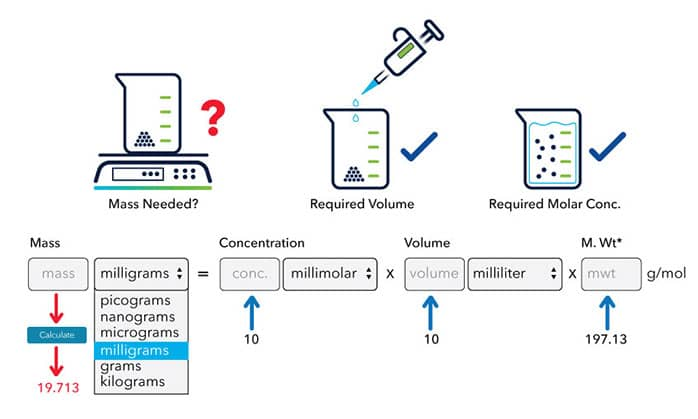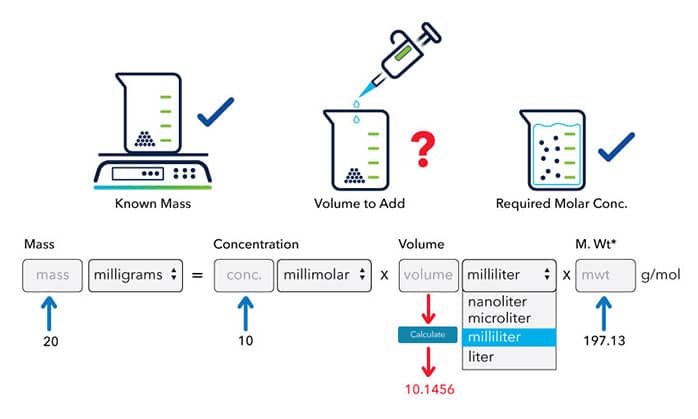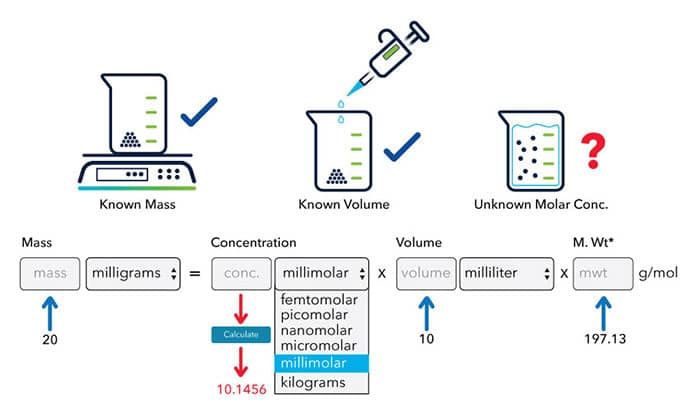# Molarity Calculator

## Calculate the Mass, Volume or Concentration Required for a Solution

The Tocris Molarity Calculator is a 3-in-1 tool which allows you to calculate the

• mass of a compound required to prepare a solution of known volume and concentration
• volume of solution required to dissolve a compound of known mass to a desired concentration
• concentration of a solution resulting from a known mass of compound in a specific volume

=
x
x
g/mol

*When preparing stock solutions always use the batch-specific molecular weight of the product found on the vial label and CoA (available online).

## The Molarity Calculator Equation

The Tocris molarity calculator is based on the following equation:

Mass (g) = Concentration (mol/L) x Volume (L) x Molecular Weight (g/mol)

## What is Molecular Weight?

Molecular mass is the mass of a single molecule of a compound. It can be calculated by summing the atomic masses of each nuclide present in the molecule and is measured in Daltons (Da or u). The atomic mass of all the known chemical elements can be found in the periodic table. For a bulk of molecules, such as a pure chemical compound supplied by Tocris, molecular weight refers to a weighted average of the molecules in the compound.

In practice, it is very difficult to accurately measure molecular weight of a compound. Molar mass and formula weight are commonly used as numerical approximations for molecular weight. Molar mass is the mass of a substance containing 1 mol of molecules; it has the unit of g/mol. The molar mass of a compound with a given chemical formula can be calculated as the sum of the atomic weights of all atoms appearing in the formula and this number is also known as the formula weight of the compound.

In general, the molecular mass, molecular weight, molar mass, or formula weight of a chemical compound can be used when using a molarity or concentration calculator for preparing a solution. In biochemistry, Daltons and g/mol (gram per mole) can be used interchangeably i.e. 1 Dalton = 1 g/mol.

## What is Molar Concentration?

Molar concentration (also known as molarity) is the amount of a solute (in moles) per unit volume of solution. It is denoted by the unit M, and 1 M = 1 mol/L. Please see the example above for how to use the Tocris molarity calculator to calculate the molarity of a solution.

To calculate how to dilute a stock solution of known concentration, please use the Tocris Dilution Calculator

To calculate the volume of a compound / protein to reconstitute your vial, please use the Tocris Reconstitution Calculator

## What is the Mass of Compound Needed to Make a Required Solution?The molecular weight of a compound is 197.13 g/mol. To make a 10 mM stock solution of this compound in 10 ml of water, the mass required can be calculated:

1. Enter 197.13 into the Molecular Weight (MW) box
2. Enter 10 into the Concentration box and select the correct unit (millimolar)
3. Enter 10 into the Volume box and select the correct unit (milliliter)
4. Press calculate
5. The answer of 19.713 mg appears in the Mass box

## How Much Solvent is Needed to Dissolve a Compound for a Required Solution?

The molecular weight of a compound is 197.13 g/mol, to make a 10 mM stock solution from 20 mg of this compound, the volume solvent can be calculated:## How to Calculate the Molarity of a Solution with Known Volume and Solute Mass?

If 20 mg of a compound, with molecular weight of 197.13 g/mol, is dissolved in 10 milliliters of a solvent. What is the molar concentration of the solution:## Supporting Scientists Since 1982

Tocris Biosciences’ catalog comprises over 4000 products with information / data covering research areas including neuroscience, cancer, endocrinology, pain and inflammation, cardiovascular, immunology, respiratory system, stem cells, COVID-19, and cell and gene therapy.

Tocris also supplies a wide range of Fluorescent dyes and probes, and high quality laboratory research reagents, such as sterile filtered DMSO (Cat. No. 3176), Bovine Serum Albumin (Cat. No. 5217), PBS tablets (Cat. No. 5564) and a list of Buffers, Solvents and Solutions for all your experiments.